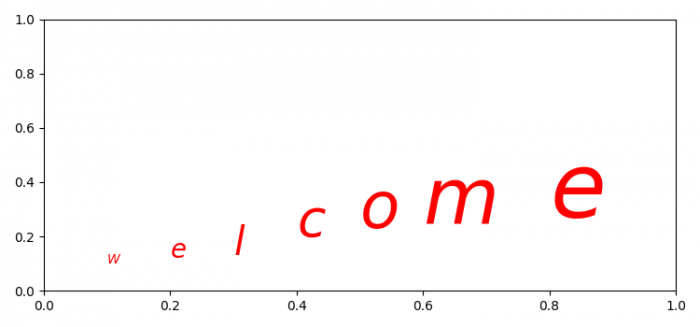# How to get different font sizes in the same annotation of Matplotlib?

MatplotlibPythonData Visualization

To add different font sizes in the same annotation method, we can take the following steps

• Make lists of x and y data points where text could be placed.
• Initialize a variable 'labels', i.e., a string.
• Make a list of sizes of the fonts.
• Use subplots() method to create a figure and a set of subplots.
• Iterate above lists and annotate each label's text and set its fontsize.
• To display the figure, use show() method.

## Example

import matplotlib.pyplot as plt
plt.rcParams["figure.figsize"] = [7.50, 3.50]
plt.rcParams["figure.autolayout"] = True
X = [0.1, .2, .3, .4, .5, .6, 0.8]
Y = [0.1, 0.12, 0.13, 0.20, 0.23, 0.25, 0.27]
labels = 'Welcome'
sizes = [10, 20, 30, 40, 50, 60, 70]
fig, ax = plt.subplots()
for x, y, label, size in zip(X, Y, labels, sizes):
ax.annotate(f"$\it{label}$", (x, y), fontsize=size, color='red')
plt.show()

## Output